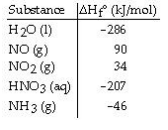Problem: Given the data in the table below, ΔH°rxn for the following reaction is __________ kJ.3NO2(g) + H2O(l) → 2HNO3 (aq) + NO (g)A) 64B) 140C) -140D) -508E) -64

FREE Expert Solution

We’re being asked to determine the standard enthalpy change of reaction. Recall that ΔH˚rxn can be calculated from the enthalpy of formation (ΔH˚f) of the reactants and products involved

We’re given the ΔH˚f for each species. The chemical equation for that reaction is:

3 NO2(g) + H2O(l) → 2 HNO(aq) + NO (g)

86% (434 ratings)
Problem Details

Given the data in the table below, ΔH°rxn for the following reaction is __________ kJ.

3NO2(g) + H2O(l) → 2HNO(aq) + NO (g)A) 64

B) 140

C) -140

D) -508

E) -64